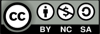# L7 Direct Democracy

## Tools For A New Political Economy

##### Example: Warren D. Smith’s Splitline Redistricting Algorithm

(Used with Permission of Warren D. Smith)

Summary:

2. Let N=A+B where A and B are as nearly equal whole numbers as possible.
(For example, 7=4+3.
More precisely, A = N/2, B=N/2.)
3. Among all possible dividing lines that split the state into two parts with population ratio A:B, choose the shortest. (Notes: since the Earth is round, when we say "line" we more precisely mean "great circle." If there is an exact length-tie for "shortest" then break that tie by using the line closest to North-South orientation, and if it's still a tie, then use the Westernmost of the tied dividing lines. "Length" means distance between the two furthest-apart points on the line, that both lie within the district being split.)
4. We now have two hemi-states, each to contain a specified number (namely A and B) of districts. Handle them recursively via the same splitting procedure.

(For additional discussion, maps and a video on this topic, visit http://rangevoting.org/GerryExamples.html)

Recursive Formulation:

Formal recursive formulation of shortest splitline districting algorithm
------------Warren D. Smith---------------------------------------------

ShortestSplitLine( State, N ){
If N=1 then output entire state as the district;
A = floor(N/2);
B = ceiling(N/2);
find shortest splitline resulting in A:B pop ratio (breaking ties,
if any, as described in notes);
Use it to split the state into the two HemiStates SA and SB;
ShortestSplitLine( SB, B );
ShortestSplitLine( SA, A );
}

---

Notes:
1. Since the Earth is round, when we say "line" we more precisely mean
"great circle." If there is an exact length-tie for "shortest" then break that tie by using
the line closest to North-South orientation, and if it's still a tie, then use the Westernmost of
the tied dividing lines.
2. If the state is convex, then a line will always split it into exactly two pieces
(each itself convex). However, for nonconvex states, a line could split it into more than
two connected pieces e.g. by "cutting off several bumps." (We expect that will occur
rarely, but it is certainly mathematically possible.) In either case the splitline's
"length" is distance between the two furthest-apart points of the line that both lie
within the region being split.
3. If anybody's residence is split in two by one of the splitlines (which would happen,
albeit very rarely) then they are automatically declared to lie in
the most-western (or if line is EW, then northern) of the two districts.
(An alternative idea would be to permit such voters to choose which district they want to be in.)

---

Example: Want N=7 districts.
Split at top level: 7 = 4+3.
Split at 2nd level: 7 = (2+2) + (1+2).
Split at 3rd level: 7 = ((1+1) + (1+1)) + ((1) + (1+1)).
result: 7 districts, all exactly equipopulous.